table div table+table div table,table.module-1{width:40.57%;float:left;padding:0}table div table+table div table a{border:0 none;text-decoration:none}table div table+table div table img{width:100%!important;border:0 none;text-decoration:none}table div table+table div table td{width:100%;padding:0 20px 20px 0}/* styles */ Welcome to August. Very excited to announce I was selected as one of the successful recipients of the English Family Foundation, Social Enterprise World Forum Australian Bursary Fund. This amazing initiative supports social enterprise leaders to attend the Social Enterprise World Forum which will be held in Addis Ababa, Ethiopia in October. I cannot wait to share my experience at the Ethical Enterprise Conference in October. Enjoy the rest of the newsletter.
 table div table+table+table div table{width:100%;padding:0}table div table+table+table div table img{width:96.23%;padding:0;float:none}table div table+table+table div table td{width:100%;padding:0 1.88% 18px}/* styles */## INSPIRING, IMPACT DRIVEN, DISRUPTIVE LEADERS

 table div table+table+table+table+table div table td,table.module-4{width:100%;padding:0}table div table+table+table+table+table div table{width:100%;float:none;margin-left:auto;margin-right:auto;padding:0}table div table+table+table+table+table div table a{border:0 none;text-decoration:none}table div table+table+table+table+table div table img{width:100%!important;border:0 none;text-decoration:none}/* styles */

# Meet the first round of speakers of this year Ethical Enterprise Conference.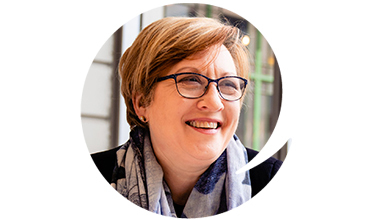Rosemary Addis [KeyNote] Impact Strategist KEYNOTE: “Scaling impact: the power of distributed leadership and collaboration in shaping an impact economy”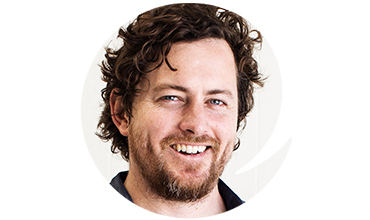Timothy O’Brien - CEO/Founder Hatched and Rooy WORKSHOP: “Collaborative tools make achieving impact a shared challenge”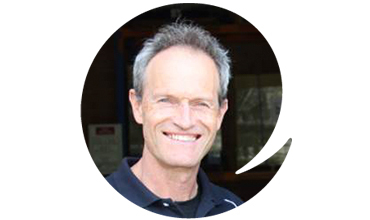Richard Etherington - Managing Director Kokonut Pacific – Niulife Pty Ltd PANEL: “Be inspired by the Winning Leaders”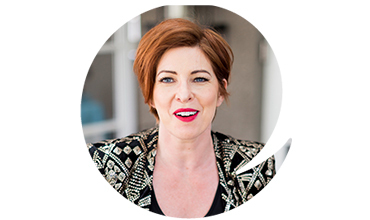Alex Tullio CEO/Founder – Alex Tullio Pty Ltd WORKSHOP: “Maximising your impact through collaboration”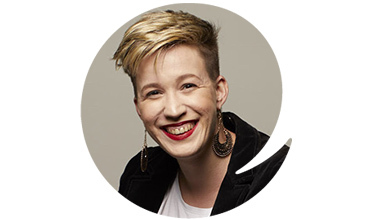Anna Gernther Co-founder and Chief Bubble Blower – PledgeMe WORKSHOP: “The future is owned by the community”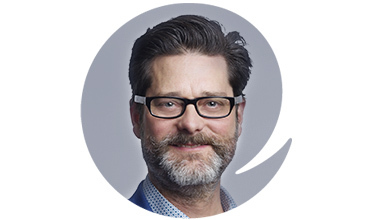Dr Mark Elliott CEO/Founder – Collabforge WORKSHOP: ” The Art & Science of Great Collaboration”
 table div table+table+table+table+table+table+table+table+table+table+table div table{width:100%;padding:0}table div table+table+table+table+table+table+table+table+table+table+table div table img{width:96.23%;padding:0;float:none}table div table+table+table+table+table+table+table+table+table+table+table div table td{width:100%;padding:0 1.88% 18px}/* styles */## Applications close on the 9th of September 2019

 table div table+table+table+table+table+table+table+table+table+table+table+table+table div table td,table.module-12{width:100%;padding:0}table div table+table+table+table+table+table+table+table+table+table+table+table+table div table{width:100%;float:none;margin-left:auto;margin-right:auto;padding:0}table div table+table+table+table+table+table+table+table+table+table+table+table+table div table a{border:0 none;text-decoration:none}table div table+table+table+table+table+table+table+table+table+table+table+table+table div table img{width:100%!important;border:0 none;text-decoration:none}/* styles */
 /* styles */ We are in the look out for Australia's most ethical enterprise. In it's 7th year, the Ethical Enterprise Award has celebrated the achievements of Australia's most inspirational for-purpose businesses striving to show that they are governed by morals over money and are making a true impact. No matter where you are in Australia, if you have been running an enterprise that has created positive social, economic or environmental impact through your business practices to local or overseas communities, we want to hear you story and celebrate your success with you. Great pool of prizes to be won to support your endeavours and great work. Thanks Dan and Richard Etherington founders of Kokonut Pacific for sending us this update on the progress of your business nearly 12 months after winning the Ethical Enterprise Award in 2018.
 table div table+table+table+table+table+table+table+table+table+table+table+table+table+table+table div table td,table.module-14{width:100%;padding:0}table div table+table+table+table+table+table+table+table+table+table+table+table+table+table+table div table{width:100%;float:none;margin-left:auto;margin-right:auto;padding:0}table div table+table+table+table+table+table+table+table+table+table+table+table+table+table+table div table a{border:0 none;text-decoration:none}table div table+table+table+table+table+table+table+table+table+table+table+table+table+table+table div table img{width:100%!important;border:0 none;text-decoration:none}/* styles */
 table div table+table+table+table+table+table+table+table+table+table+table+table+table+table+table+table+table div table{width:100%;padding:0}table div table+table+table+table+table+table+table+table+table+table+table+table+table+table+table+table+table div table img{width:96.23%;padding:0;float:none}table div table+table+table+table+table+table+table+table+table+table+table+table+table+table+table+table+table div table td{width:100%;padding:0 1.88% 18px}/* styles */## Start-up, early stage social entrepreneurs, apply before the 9th of September

 table div table+table+table+table+table+table+table+table+table+table+table+table+table+table+table+table+table+table+table div table td,table.module-18{width:100%;padding:0}table div table+table+table+table+table+table+table+table+table+table+table+table+table+table+table+table+table+table+table div table{width:100%;float:none;margin-left:auto;margin-right:auto;padding:0}table div table+table+table+table+table+table+table+table+table+table+table+table+table+table+table+table+table+table+table div table a{border:0 none;text-decoration:none}table div table+table+table+table+table+table+table+table+table+table+table+table+table+table+table+table+table+table+table div table img{width:100%!important;border:0 none;text-decoration:none}/* styles */
 /* styles */ We know there are many of you out there with an idea or a concept that will have the potential to challenge the norm, to make way for a better and more sustainable future. Good ideas are not enough, you need to put it out there and let the world know about it. The Early Ethical Entrepreneur Pitch Competition provides a perfect forum to share your idea to a network of people that are keen not only to hear your idea but also to help you on your journey to make it happen. APPLICATIONS CLOSE ON THE 9TH OF SEPTEMBER Astaria Geor founder of Ecopact Grocery was one of the 2018 Early Ethical Entrepreneur Pitch Competition Finalists. Ecopact was just a dream when they pitched their idea in front of a room full of people at the Ethical Enterprise Conference, now it has become a reality.
 table.module-20{width:74.53%;padding:0}table div table+table+table+table+table+table+table+table+table+table+table+table+table+table+table+table+table+table+table+table+table div table{width:74.53%;float:none;margin-left:auto;margin-right:auto;padding:0}table div table+table+table+table+table+table+table+table+table+table+table+table+table+table+table+table+table+table+table+table+table div table a{border:0 none;text-decoration:none}table div table+table+table+table+table+table+table+table+table+table+table+table+table+table+table+table+table+table+table+table+table div table img{width:100%!important;border:0 none;text-decoration:none}table div table+table+table+table+table+table+table+table+table+table+table+table+table+table+table+table+table+table+table+table+table div table td{width:100%;padding:0}/* styles */
 table div table+table+table+table+table+table+table+table+table+table+table+table+table+table+table+table+table+table+table+table+table+table+table div table{width:100%;padding:0}table div table+table+table+table+table+table+table+table+table+table+table+table+table+table+table+table+table+table+table+table+table+table+table div table img{width:96.23%;padding:0;float:none}table div table+table+table+table+table+table+table+table+table+table+table+table+table+table+table+table+table+table+table+table+table+table+table div table td{width:100%;padding:0 1.88% 18px}/* styles */## STUDENT PRICING NOW AVAILABLE

 table div table+table+table+table+table+table+table+table+table+table+table+table+table+table+table+table+table+table+table+table+table+table+table+table+table div table td,table.module-24{width:100%;padding:0}table div table+table+table+table+table+table+table+table+table+table+table+table+table+table+table+table+table+table+table+table+table+table+table+table+table div table{width:100%;float:none;margin-left:auto;margin-right:auto;padding:0}table div table+table+table+table+table+table+table+table+table+table+table+table+table+table+table+table+table+table+table+table+table+table+table+table+table div table a{border:0 none;text-decoration:none}table div table+table+table+table+table+table+table+table+table+table+table+table+table+table+table+table+table+table+table+table+table+table+table+table+table div table img{width:100%!important;border:0 none;text-decoration:none}/* styles */
 /* styles */ We have received a considerable amount of interest from the student community across Australia and we have now been able to generate a student ticket price for the "Conference Only" package. This is limited to 30 tickets, so if you are currently studying and have a student ID, book now before tickets run out.
 table div table+table+table+table+table+table+table+table+table+table+table+table+table+table+table+table+table+table+table+table+table+table+table+table+table+table+table+table div table{width:100%;padding:0}table div table+table+table+table+table+table+table+table+table+table+table+table+table+table+table+table+table+table+table+table+table+table+table+table+table+table+table+table div table img{width:96.23%;padding:0;float:none}table div table+table+table+table+table+table+table+table+table+table+table+table+table+table+table+table+table+table+table+table+table+table+table+table+table+table+table+table div table td{width:100%;padding:0 1.88% 18px}/* styles */## Book your complimentary ticket to "The Collaborator"

 table.module-29{width:99.62%;padding:0}table div table+table+table+table+table+table+table+table+table+table+table+table+table+table+table+table+table+table+table+table+table+table+table+table+table+table+table+table+table+table div table{width:99.62%;float:none;margin-left:auto;margin-right:auto;padding:0}table div table+table+table+table+table+table+table+table+table+table+table+table+table+table+table+table+table+table+table+table+table+table+table+table+table+table+table+table+table+table div table a{border:0 none;text-decoration:none}table div table+table+table+table+table+table+table+table+table+table+table+table+table+table+table+table+table+table+table+table+table+table+table+table+table+table+table+table+table+table div table img{width:100%!important;border:0 none;text-decoration:none}table div table+table+table+table+table+table+table+table+table+table+table+table+table+table+table+table+table+table+table+table+table+table+table+table+table+table+table+table+table+table div table td{width:100%;padding:0}/* styles */

# Do you run a business in the City of Whittlesea?

Moral Fairground supported by City of Whittlesea, is inviting 16 to 20 businesses to apply for a series of workshops aimed at local businesses that are focused on community benefit. The aim of this program is to undertake capacity building for local businesses with purpose and ultimately to form a community of ethical businesses that support and work with each other to deliver better social outcomes.
The program aims to bring together social enterprises, and community-focused businesses, as well as individuals and businesses that want to explore how to achieve positive social and environmental outcomes through business.

## DATES: 4th Sept 2019; 11st Sept 2019; 25th Sept 2019 and 2nd Oct 2019

Details here.

 table div table+table+table+table+table+table+table+table+table+table+table+table+table+table+table+table+table+table+table+table+table+table+table+table+table+table+table+table+table+table+table+table+table div table{width:100%;padding:0}table div table+table+table+table+table+table+table+table+table+table+table+table+table+table+table+table+table+table+table+table+table+table+table+table+table+table+table+table+table+table+table+table+table div table img{width:96.23%;padding:0;float:none}table div table+table+table+table+table+table+table+table+table+table+table+table+table+table+table+table+table+table+table+table+table+table+table+table+table+table+table+table+table+table+table+table+table div table td{width:100%;padding:0 1.88% 18px}/* styles */table.module-34{width:99.62%;padding:0}table div table+table+table+table+table+table+table+table+table+table+table+table+table+table+table+table+table+table+table+table+table+table+table+table+table+table+table+table+table+table+table+table+table+table+table div table{width:99.62%;float:none;margin-left:auto;margin-right:auto;padding:0}table div table+table+table+table+table+table+table+table+table+table+table+table+table+table+table+table+table+table+table+table+table+table+table+table+table+table+table+table+table+table+table+table+table+table+table div table a{border:0 none;text-decoration:none}table div table+table+table+table+table+table+table+table+table+table+table+table+table+table+table+table+table+table+table+table+table+table+table+table+table+table+table+table+table+table+table+table+table+table+table div table img{width:100%!important;border:0 none;text-decoration:none}table div table+table+table+table+table+table+table+table+table+table+table+table+table+table+table+table+table+table+table+table+table+table+table+table+table+table+table+table+table+table+table+table+table+table+table div table td{width:100%;padding:0}/* styles */
 /* styles */ You can join the Moral Fairground membership at any time to get access to discounts, gain exposure through our range of events and programs or share your initiatives via our networks. Join us today.
 table div table+table+table+table+table+table+table+table+table+table+table+table+table+table+table+table+table+table+table+table+table+table+table+table+table+table+table+table+table+table+table+table+table+table+table+table+table div table{width:100%;padding:0}table div table+table+table+table+table+table+table+table+table+table+table+table+table+table+table+table+table+table+table+table+table+table+table+table+table+table+table+table+table+table+table+table+table+table+table+table+table div table img{width:96.23%;padding:0;float:none}table div table+table+table+table+table+table+table+table+table+table+table+table+table+table+table+table+table+table+table+table+table+table+table+table+table+table+table+table+table+table+table+table+table+table+table+table+table div table td{width:100%;padding:0 1.88% 18px}/* styles */## GoodWill Wine

David began Goodwill Wine after losing most of what he owned in the Black Saturday bushfires. With a strong desire to pay forward the help he received, he took the \$15,000 given to him through the Red Cross Bushfire Appeal and instead of replacing what was lost, began sourcing high quality wines, built a website, and started passing on 50% of his profit to charity. Fast forward ten years, some recent angel investment and a team made up largely from the long term unemployed as well as the NDIS, and Goodwill Wine has grown rapidly; tripling its turnover in 2019 and paying forward over \$275,000.
Buy Wines and support good causes.

 table div table+table+table+table+table+table+table+table+table+table+table+table+table+table+table+table+table+table+table+table+table+table+table+table+table+table+table+table+table+table+table+table+table+table+table+table+table+table+table+table div table{width:100%;padding:0}table div table+table+table+table+table+table+table+table+table+table+table+table+table+table+table+table+table+table+table+table+table+table+table+table+table+table+table+table+table+table+table+table+table+table+table+table+table+table+table+table div table img{width:96.23%;padding:0;float:none}table div table+table+table+table+table+table+table+table+table+table+table+table+table+table+table+table+table+table+table+table+table+table+table+table+table+table+table+table+table+table+table+table+table+table+table+table+table+table+table+table div table td{width:100%;padding:0 1.88% 18px}/* styles */## The fifth Melbourne Soup

When: Sunday the 15th of September 2019
Cost: \$15.00
Location: Youthworx in Brunswick
Booking: HERE
Melbourne SOUP returns for a fifth event on the 15th of September at youthworx in Brunswick. Attendees pay \$15 and hear 4 presentations on ideas that address a social or environmental issue. Everyone then enjoys a bowl of soup and some live music, while voting for one of the presentations. The presenter who receives the most votes takes home the majority of the ticket money raised, and returns to the next SOUP event to update the community on their progress. Expect a night filled with good causes, good food and good people. All the details, including how to apply to present, are available via the Facebook event.

## How to become a Bcorp

Business plays a critical role in solving the problems our planet faces. B Corporation is a global movement of business as a force for good. It is also a certification for ethical business practice, looking at environmental and social performance indicators as well as profit. The growth of the purpose driven business movement globally reflects trends in values driven consumerism.
 table div table+table+table+table+table+table+table+table+table+table+table+table+table+table+table+table+table+table+table+table+table+table+table+table+table+table+table+table+table+table+table+table+table+table+table+table+table+table+table+table+table+table+table+table div table{width:100%;padding:0}table div table+table+table+table+table+table+table+table+table+table+table+table+table+table+table+table+table+table+table+table+table+table+table+table+table+table+table+table+table+table+table+table+table+table+table+table+table+table+table+table+table+table+table+table div table img{width:96.23%;padding:0;float:none}table div table+table+table+table+table+table+table+table+table+table+table+table+table+table+table+table+table+table+table+table+table+table+table+table+table+table+table+table+table+table+table+table+table+table+table+table+table+table+table+table+table+table+table+table div table td{width:100%;padding:0 1.88% 18px}/* styles */